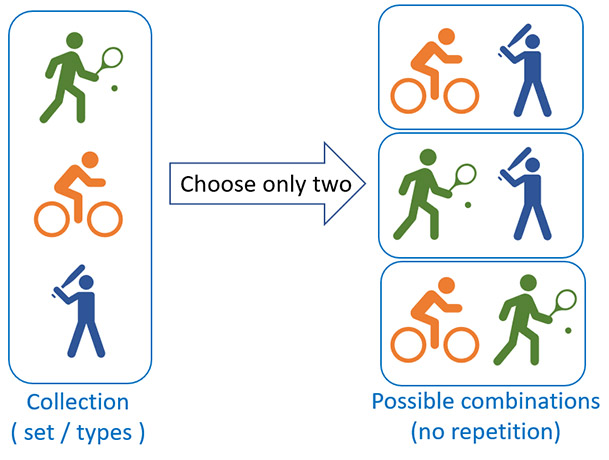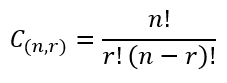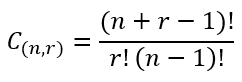# Combination Calculator

Use this nCr calculator to easily calculate the number of combinations given a set of objects (types) and the number you need to draw from the set. N choose K calculator online to calculate how many combinations with N numbers are possible.

### Calculation results

10 Combinations
Share calculator:

Embed this tool:
get code

## What is a combination?

A combination is a way to select a part of a collection, or a set of things in which the order does not matter and it is exactly these cases in which our combination calculator can help you. For example, if you want a new laptop, a new smartphone and a new suit, but you can only afford two of them, there are three possible combinations to choose from: laptop + smartphone, smartphone + suit, and laptop + suit. The order in which you combine them doesn't matter, as you will buy the two you selected anyways. Combinations come up a lot when you need to estimate the number of possible connections or groupings between things or people.

Calculating combinations is useful in games of chance like lottery, poker, bingo, and other types of gambling or games in which you need to know your chance of success or failure (odds), which is usually expressed as a ratio between the number of combinations in play that will result in you winning divided by the number of possible combinations that will result in your losing.

For example, the odds of winning the US Powerball lottery jackpot are about 1 in 292 million (1/292,201,338) where 292,201,338 is total number of possible combinations. The order in most lottery draws does not matter. If we examine the poker example further: a poker hand can be described as a 5-combination of cards from a 52-card deck. The 5 cards of the hand are all distinct, and the order of cards in the hand does not matter so it is a combinatorial problem. Using our combination calculator, you can calculate that there are 2,598,960 such combinations possible, therefore the chance of drawing a particular hand is 1 / 2,598,960.

Here is a more visual example of how combinations work. Say you have to choose two out of three activities (a 3 choose 2 problem): cycling, baseball and tennis, the possible combinations would look like so:Combination calculations play a part in statistics, problem solving and decision-making algorithms, and others.

## How to calculate combinations?

There are two formulas for calculating the number of possible combinations in an "n choose k" a.k.a. "n choose r" scenario, depending on whether repetition of the chosen elements is allowed or not. In both equations "!" denotes the factorial operation: multiplying the sequence of integers from 1 up to that number. For example, a factorial of 4 is 4! = 4 x 3 x 2 x 1 = 24.

### Combination formula without repetition

To calculate the number of possible combinations of r non-repeating elements from a set of n types of elements, the formula is:The above equation therefore expresses the number of ways for picking r unique unordered outcomes from n possibile entities and is often referred to as the nCr formula.

### Formula for possible combinations with repetition

If the elements can repeat in the combination, the respective equation is:The result is the number of all possible ways of choosing r non-unique elements from a set of n elements. In some versions of the above formulas r is replaced by k with no change in their outcome or interpretation.

## Combinations with repetition

In some cases, repetition of the same element is desired in the combinations. For example, if you are trying to come up with ways to arrange teams from a set of 20 people repetition is impossible since everyone is unique, however if you are trying to select 2 fruits from a set of 3 types of fruit, and you can select more than one from each type, then it is a problem with repetition. The formula for its solution is provided above, but in general it is more convenient to just flip the "with repetition" checkbox in our combination calculator and let us do the work for you.

## N choose K problems with solutions

Often encountered problems in combinatorics involve choosing k elements from a set of n, or the so-called "n choose k" problems, also known as "n choose r". Here we will examine a few and work through their solutions. These can all be verified using our ncr formula calculator above.

### How many combinations with N numbers?

In the simplest version of these problems N equals K (or R) in which it is often implied that repetition is allowed, otherwise the answer is always one. If repetition is allowed, the answer is can be obtained by solving the equation (2 · n - 1)! / (n! · (n - 1)!). For example, if the task is to find how many combinations are possible with 4 numbers, compute (2 · 4 - 1)! = 7! = 7 · 6 · 5 · 4 · 3 · 2 · 1 = 5040 / (24 · 6) = 5040 / 144 = 35.

### 3 choose 2

What if one is asked to determine how many unique combinations of two numbers are possible if one is choosing from a total of three? The answer, using the ncr formula without repetition above is simply: 3! / (2! · (3 - 2)!) = 3! / (2! · 1!) = 3 · 2 · 1 / (2 · 1 · 1) = 6 / 2 = 3. With 3 choose 2 there are just 3 possible combinations.

### 4 choose 2

What if we are choosing 2 out of 4 items, no repetition allowed? Using the same formula and replacing N and R, we get the answer 4! / (2! · (4 - 2)!) = 24 / (2! · 2!) = 24 / 4 = 6 ways to choose two unique elements out of a total of four.

### 4 choose 3

To calculate how many combinations of three out of four items can be chosen without repeating an item, use the ncr formula and replace to get 4! / (3! · (4 - 3)!) = 24 / (3! · 1!) = 24 / 6 = 4. Note that this is less than if you were choosing two out of four as in the previous example.

## N choose K table

Here is a table with solutions to commonly encountered combination problems known as n choose k or n choose r, depending on the notation used. Solutions are provided both with and without repetition.

n choose k table
CombinationCombinations without repetitionCombinations with repetition
2 choose 1 2 2
2 choose 2 1 3
3 choose 1 3 3
3 choose 2 3 6
3 choose 3 1 10
4 choose 1 4 4
4 choose 2 6 10
4 choose 3 4 20
4 choose 4 1 35
5 choose 1 5 5
5 choose 2 10 15
5 choose 3 10 35
5 choose 4 5 70
5 choose 5 1 126
6 choose 1 6 6
6 choose 2 15 21
6 choose 3 20 56
6 choose 4 15 126
6 choose 5 6 252
6 choose 6 1 462
7 choose 1 7 7
7 choose 2 21 28
7 choose 3 35 84
7 choose 4 35 210
7 choose 5 21 462
7 choose 6 7 924
7 choose 7 1 1,716
8 choose 4 70 330
10 choose 4 210 715

For other solutions, simply use the nCr calculator above.

Examining the table, three general rules can be inferred:

• Rule #1: For combinations without repetition, the highest number of possibilities exists when r = n / 2 (k = n/2 if using that notation). For example, if choosing out of six items, one has the most possible combinations when r = 6 / 2 = 3 (k = 3 if using k instead of r).
• Rule #2: with repetition, the number of possible combinations increases the closer r is to n (or k is to n in that notation).
• Rule #3: without repetition, if n = r (or n = k), there is just a single possible draw.

## Combinations vs permutations

The difference between combinations and permutations is that while when counting combinations we do not care about the order of the things we combine with permutations the order matters. Permutations are for ordered lists, while combinations are for unordered groups. For example, if you are thinking of the number of combinations that open a safe or a briefcase, then these are in fact, permutations, since changing the order of the numbers or letters would result in an invalid code. If, however, you are thinking of the number of ways to combine your dresses with your shoes or your ties with your suits, then order doesn't matter, since the end result of choosing the tie first and the suit second is the same as choosing the suit first and the tie second.

A lot of times in common usage people call permutations"combinations" incorrectly. For example, a lock combination is in fact a permutation. In another example - if you want to estimate how many computing hours you need to brute force a hashed password you calculate the number of permutations, not the number of combinations.

#### Cite this calculator & page

If you'd like to cite this online calculator resource and information as provided on the page, you can use the following citation:
Georgiev G.Z., "Combination Calculator", [online] Available at: https://www.gigacalculator.com/calculators/combination-calculator.php URL [Accessed Date: 01 Apr, 2023].